Download Presentation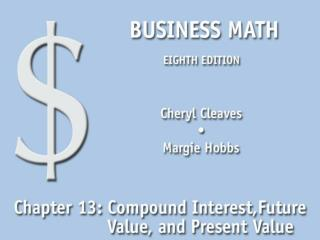13.1 Compound Interest and Future Value

# 13.1 Compound Interest and Future Value - PowerPoint PPT Presentation

13.1 Compound Interest and Future Value. Find the future value and compound interest by compounding manually. Find the future value and compound interest by using a \$1.00 future value table. Find the future value and compound interest using a formula (optional).I am the owner, or an agent authorized to act on behalf of the owner, of the copyrighted work described.
Download Presentation## 13.1 Compound Interest and Future Value

An Image/Link below is provided (as is) to download presentation

Download Policy: Content on the Website is provided to you AS IS for your information and personal use and may not be sold / licensed / shared on other websites without getting consent from its author.While downloading, if for some reason you are not able to download a presentation, the publisher may have deleted the file from their server.

- - - - - - - - - - - - - - - - - - - - - - - - - - E N D - - - - - - - - - - - - - - - - - - - - - - - - - -
Presentation Transcript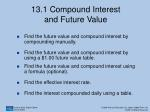13.1 Compound Interest and Future Value
• Find the future value and compound interest by compounding manually.
• Find the future value and compound interest by using a \$1.00 future value table.
• Find the future value and compound interest using a formula (optional).
• Find the effective interest rate.
• Find the interest compounded daily using a table.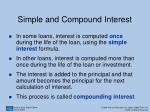Simple and Compound Interest
• In some loans, interest is computed once during the life of the loan, using the simpleinterest formula.
• In other loans, interest is computed more than once during the life of the loan or investment.
• The interest is added to the principal and that amount becomes the principal for the next calculation of interest.
• This process is called compounding interest.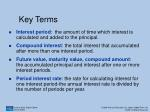Key Terms
• Interest period: the amount of time which interest is calculated and added to the principal.
• Compound interest: the total interest that accumulated after more than one interest period.
• Future value, maturity value, compound amount: the accumulated principal and interest after one or more interest periods.
• Period interest rate: the rate for calculating interest for one interest period-the annual interest rate is divided by the number of periods per year.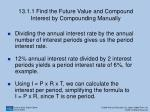13.1.1 Find the Future Value and Compound Interest by Compounding Manually
• Dividing the annual interest rate by the annual number of interest periods gives us the period interest rate.
• 12% annual interest rate divided by 2 interest periods yields a period interest rate of 6%, for example.
• Using I = P x R x T, we can calculate the interest per period, simplifying the formula to I = P x R, since T is one period.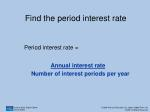Find the period interest rate

Period interest rate =

Annual interest rate

Number of interest periods per year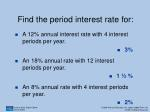Find the period interest rate for:
• A 12% annual interest rate with 4 interest periods per year.
• 3%
• An 18% annual rate with 12 interest periods per year.
• 1 ½ %
• An 8% annual rate with 4 interest periods per year.
• 2%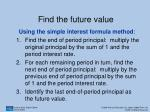Find the future value

Using the simple interest formula method:

• Find the end of period principal: multiply the original principal by the sum of 1 and the period interest rate.
• For each remaining period in turn, find the next end of period principal: multiply by the previous end of period principal by the sum of 1 and the period interest rate.
• Identify the last end-of-period principal as the future value.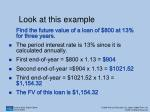Look at this example

Find the future value of a loan of \$800 at 13% for three years.

• The period interest rate is 13% since it is calculated annually.
• First end-of-year = \$800 x 1.13 = \$904
• Second end-of-year =\$904 x 1.13 = \$1021.52
• Third end-of-year = \$1021.52 x 1.13 = \$1,154.32
• The FV of this loan is \$1,154.32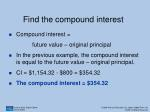Find the compound interest
• Compound interest =

future value – original principal

• In the previous example, the compound interest is equal to the future value – original principal.
• CI = \$1,154.32 - \$800 = \$354.32
• The compound interest = \$354.32Compare the compound interest amount to the simple interest
• CI = \$354.32
• Simple interest for the same loan would be:
• I = PRT
• I = \$800 x 0.13 x 3 = \$312.00
• Simple interest would be \$312.00
• The difference between compound interest and simple interest for this loan = \$354.32 - \$312
• The difference is \$43.32Find the FV of an investment
• Principal = \$10,000
• 8% annual interest rate, compounded semi-annually
• Find the FV at the end of three years.
• Find the period interest rate: 8% ÷ 2 = 4%
• Determine number of periods: 3 x 2 = 6
• Calculate each end-of-period principal.
• Period 1 = 10,000 x 1.04 = \$10,400Find the FV of an investment
• Second end-of-period principal = \$10,400 x 1.04 = \$10,816
• Calculate each end-of-principal through the sixth end-of-period principal.
• What is the final end-of-principal amount?
• \$12,653.1913.1.2 Using a \$1.00 FV Table
• Since it would be tedious and time-consuming to calculate a large number of periods with the previous method, we can use Table 13-1, which is the future value or compound amount of \$1.00.
• Find the number of periods and the rate per period to identify the value by which the principal is multiplied.Look at this example
• Using Table 13-1, find the compound interest on \$500 for six years compounded annually at 8%.
• Determine the number of periods: 6
• Determine the interest rate per period: 8%
• Locate the value in the intersecting cell: 1.58687
• Multiply the principal, \$500, x 1.58687 = \$793.44
• The FV of the loan is \$793.44.
• Compound interest = \$793.44- \$500 = \$293.44Try this example
• Using Table 13-1, find the future value and compound interest on \$2,000 invested for four years compounded semiannually at 8%.
• FV = \$2,737.14
• CI = \$737.14
• What would the simple interest be for the same loan?
• \$64013.1.3 Find the Future Value and Compound Interest Using a Formula (optional)
• The future value formula is:

FV = where FV is the future value, P is the principal, R is the period interest rate, and N is the number of periods.

• The formula for finding future value will require a calculator that has a power function.Try this example
• Find the future value and compound interest of a 3-year \$5,000 investment that earns 6% compounded monthly.
• FV =
• FV =
• FV = \$5,983.40
• CI = \$5,983.40 – \$5,000 = \$983.4013.1.4 Find the Effective Interest Rate
• Effective interest rate is also called the annual percentage yield or APY when identifying rate of earning on an investment.
• It is called APR, annual percentage rate, when identifying the rate of interest on a loan.
• Effective rate: the equivalent simple interest rate that is equivalent to a compound rateLook at this example
• Marcia borrowed \$600 at 10% compounded semiannually. What is the effective interest rate?
• Using the manual compound interest method:
• Period rate interest = 10% / 2 = 5% = 0.05
• First end-of-period principal = \$600 x 1.05 = \$630
• Second end-of-principal = \$630 x 1.05 =\$661.50
• Compound interest after first year = \$61.50Effective interest rate

Annual effective interest rate = \$61.50\$600

Multiplied by 100%

= 0.1025 x 100%

= 10.25%

Using the table method (Table 13-1):

The table value is 1.10250. Subtract 1.00 and multiply by 100%. The effective rate is 10.25%13.1.5 Find the Interest Compounded Daily Using a Table
• Table 13-2 gives compound interest for \$100 compounded daily (using 365 days as a year.)
• Pay attention to the table value given. Table 13-2 uses \$100 as the principal amount; other tables may use \$1, \$10 or other amounts.
• Using Table 13-2 is exactly like using Table 11-2 which gives the simple interest on \$100.Look at this example
• Find the interest on \$800 at 7.5% annually, compounded daily for 28 days.
• Divide the principal by \$100 as you are using Table 13-2. [\$800 ÷ 100 = 8]
• Find the corresponding value by intersecting the number of days (28) and annual interest rate (7.5%) =0.5769413
• Multiply this value by 8 = \$4.62
• The compounded interest is \$4.62Try this example
• Find the interest on \$1,000 for 30 days compounded at a 6% annual rate.
• Answer:Divide \$1,000 ÷ 100 = 10Locate the cell where 30 days and 6% intersect to determine the value: 0.4943279Multiply by 10.The interest is\$4.9413.2 Present Value
• Find the present value based on annual compounding for one year.
• Find the present value using a \$1.00 present value table.
• Find the present value using a formula (optional).• Suppose you want to go on a long vacation in a couple of years…or pay for your child’s college education. How much money would you have to invest right now to be able to pay for it?
• Using the concepts of compound interest, you can determine amounts needed now to cover expenses in the future.
• The amount of money you set aside now is called present value.Present value
• The simplest case would be annual compounding interest for one year: the number of interest periods is 1 and the period interest rate is the annual interest rate.
• Principal (present value) = future value

1 + annual interest rate*

* denotes decimal equivalentLook at this example
• Find the amount of money that The 7th Inning needs to set aside today to ensure that \$10,000 will be available to buy a new large screen plasma television in one year if the annual interest rate is 4% compounded annually.
• PV = 10,000 1.04 = \$9,615.38
• An investment of \$9,615.38 at 4% would have a value of \$10,000 in one year.Try these examples
• Calculate the amount of money needed now to purchase a laptop computer and accessories valued at \$2,000 in a year if you invest the money at 6%.
• \$1,886.79
• John wants to replace a tool valued at \$150 in a year. How much money will he have to put into a savings account that pays 3% annual interest?
• \$145.6313.2.2. Use a \$1.00 Present Value Table
• Using a present value table is the most efficient way to calculate the money needed now for a future expense or investment.
• Table 13-3 shows the present value of \$1.00 at different interest rates for different periods.How to use the table
• Find the number of interest periods: multiply the time period in years by number of interest periods per year.Interest periods = number of years x number of interest periods per year
• Find the interest rate: divide the annual interest rate by the number of interest periods per year.Period interest rate = annual interest rate Number of interest periods per yearUsing the table (continued)

3. Select the periods row corresponding to the number of interest periods.

4. Select the rate per period column corresponding to the period interest rate.

5. Locate the value in the cell where the periods row intersects the rate-per-period column.

6. Multiply the future value by value from step 5.Look at this example
• The 7th Inning needs \$35,000 in 4 years to buy new framing equipment. How much should be invested at 4% interest compounded annually?
• 4 periods at 4% shows a value of 0.85480
• Multiply this value by \$35,000
• The result is \$29,918
• They must invest \$29,918 at 4% compounded annually for four years to have \$35,000Try these examples
• How much money would you have to invest for 5 years at 6% paid semi-annually to make a down payment of \$20,000 on a house?
• \$14,881.80
• How much money would you have to invest for 3 years at 10% paid semi-annually to purchase an automobile that costs \$20,000?
• \$14,924.4013.2.3 Find the Present Value Using a Formula (optional)
• The present value formula is:

PV =

where PV is the present value, FV is the future value, R is the period interest rate, and N is the number of periods.Try this example
• Find the present value required at 5.2% compounded monthly to total \$8,000 in three years.
• PV =
• Period int. rate = 5.2%/12 = .0043333333
• PV = = \$6,846.78